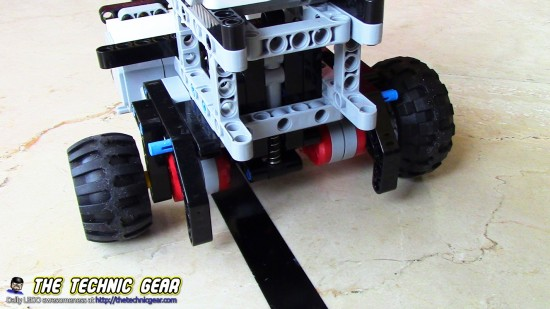# What happens when a Robot has different size wheels?

This article is another spin-off of the Differential-drive robot calibration. If you remember the article at the end you found that wheels are most of the times of different sizes with differences of around 0.01% but that are big enough to affect movement over 1 meter. So…

What happens when you move one meter straight with different wheels?So the best way of understanding the problem was to force a big wheel difference so I just replaced with two different wheels: one of 56mm and another of 44mm of diameter. So let’s begin to see what happens with the simplest case.

## Differential drive movement theory

If you need to know a bit of the Maths behind straight line movemeny, I explained them in this short video.

## Different size wheels and movement using biggest wheel diameter

The simplest approach is using the biggest wheel diameter and cross your fingers.
The code is pretty much like this:

```      double maxWheel=leftWheel;
if(rightWheel>leftWheel) {
maxWheel=rightWheel;
}

degrees=(int)((length/(maxWheel*Math.PI))*360);

left.rotate(degrees,true);
right.rotate(degrees);
left.waitComplete();
```

As you can see on the video the robot steers clearly to the side with the smallest wheel, and that is because the biggest wheels is moving more distance that the smallest one.

## Different size wheels and movement using average wheel diameter

We can try to compute the average diameter and move the average diameter… will it be useful?
The code is pretty much like this:

```      double meanWheel=(leftWheel+rightWheel)/2.0;

degrees=(int)((length/(meanWheel*Math.PI))*360);

left.rotate(degrees,true);
right.rotate(degrees);
left.waitComplete();
```

Again, as you can see in the video it still moves to the side with the smallest wheel for the very same reasons that the above case.

## Using different amount of rotations for each motor

The problem is that the smallest wheel runs less distance than the big wheel so we could try to make each wheel rotate a different amount of degrees with a code like this.

```      degrees=(int)((length/(leftWheel*Math.PI))*360);
left.rotate(degrees,true);

degrees=(int)((length/(rightWheel*Math.PI))*360);
right.rotate(degrees);

left.waitComplete();
```

Now both wheels will move one meter… but as you can see on the video it is still not enough. Why? Because despite the fact that both wheels moves the same amount of distance, they do it at different speed.

## Using different speed for each wheel

Ok, if you are still here you should have realized that we need to apply different speed to each wheel… but how do we set speed?

v = d/t

So we can write

vl = dl * π/t
vr = dr * π/t

As we want them to arrive at the same time, t is the same for both expressions so we can write:

dl / vl = dr / vr

We set vl to any value we want and then we can define vr as

vr = dt * vl / dl

So, this is the code:

```    int lspeed=400;
left.setSpeed(lspeed);
int rspeed=(int)(lspeed*leftWheel/rightWheel);
right.setSpeed(rspeed);

degrees=(int)((length/(leftWheel*Math.PI))*360);
left.rotate(degrees,true);

degrees=(int)((length/(rightWheel*Math.PI))*360);
right.rotate(degrees);

left.waitComplete();
```

So you can see the result on the video and … WTF!!! It doesn’t work. Why? WHY! oh, Why!? Look again the video and see how the biggest wheel reaches its top speed sooner than the smaller resulting in a small but noticeable turn.

## Using different accelerations for each wheel

So, in our last step to solve the problem we are going to calculate the new acceleration for each wheel. Again, we can define acceleration as:

a= Δv / Δt

If we consider the time from zero to top speed we get:

al = vl / t
ar = vr / t

As we want to reach the top speed at the same time we can write

vl / al = vr / ar

And as we did before… we set the left acceleration to some reasonable value and calculate right one with

ar = vr * al / vl

So we get this code…

```  lspeed=400;
left.setSpeed(lspeed);
rspeed=(int)(lspeed*leftWheel/rightWheel);
right.setSpeed(rspeed);

int lacceleration=400;
left.setAcceleration(lacceleration);
int racceleration=(int)(lacceleration*rspeed/(double)lspeed);
right.setAcceleration(racceleration);

degrees=(int)((length/(leftWheel*Math.PI))*360);
left.rotate(degrees,true);

degrees=(int)((length/(rightWheel*Math.PI))*360);
right.rotate(degrees);

left.waitComplete();
```

And as you can see on the video it does work! Yes!

Just import these two files in a leJOS Eclipse and you are done. Or simply put them on the same folder and make it an Eclipse source folder.

## Miguel says…

It is really cool to see the asymmetric robot moves straight and as I have been writing lately I wasn’t expecting something so simple to be so hard. Good luck implementing this using EV3 software…

### 1 thought on “What happens when a Robot has different size wheels?”

1.Robert

Hi Miguel,

any chance to use this code for Mindstorm standard programming surface, not LeJos?

Regards Robert